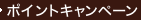•### Quantum Mechanics

• ただいまウェブストアではご注文を受け付けておりません。 ⇒古書を探す
• 製本 Hardcover:ハードカバー版／ページ数 544 p.
• 言語 ENG
• 商品コード 9780131461000
• DDC分類 530.12

### Full Description

With clear discussion and numerous problems, this text is the first to adequately and comprehensively cover all the subjects of quantum mechanics. It carefully and thoroughly discusses symmetries--especially rotation symmetry, transition theory, the theory of the quantized electromagnetic field, and relativistic wave equations. For physicists, engineers, and chemists.

### Contents

1. Classical Mechanics. 2. Fundamentals of Quantum Mechanics. 3. Stationary States. 4. Symmetry Transformations on States. 5. Symmetry Transformations on Operators. 6. Interlude. 7. Approximation Methods for Bound States. 8. Potential Scattering. 9. Transitions. 10. Further Topics in Quantum Dynamics. 11. The Quantized Electromagnetic Field. 12. Relativistic Wave Equations. 13. Identical Particles. Appendix A. Mathematical Tools. Appendix B. Rotation Matrices. Appendix C. SU 3. Appendix D. References. Index.